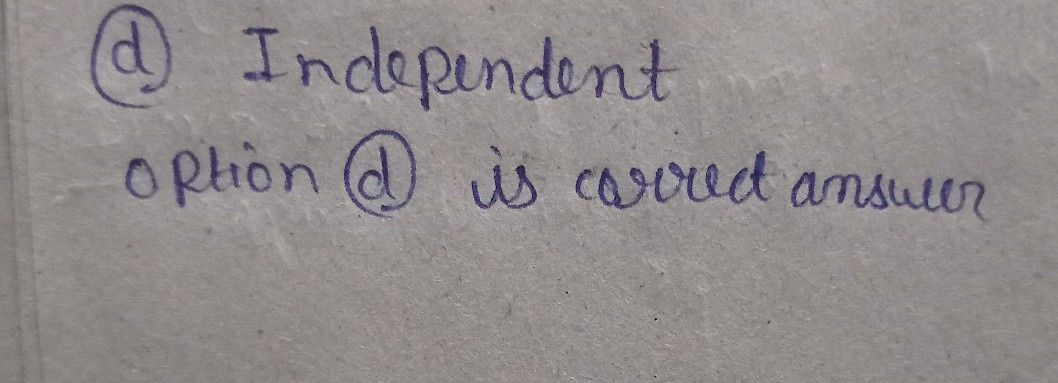Symbol
ProblemWhat do you call a relation where each element in the domain is related to only one value $1$ in the $1$ range $\right)y$ some rules? $a$ Function c. Domain $1\right)$ Range d. Independent
10th-13th grade
Other
Search count: 105
SolutionQanda teacher - Anshulplease give me review by 5 ?